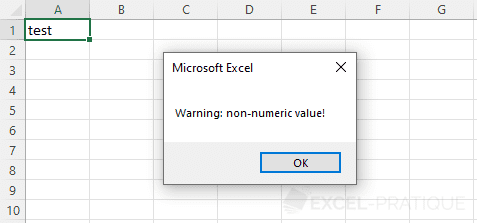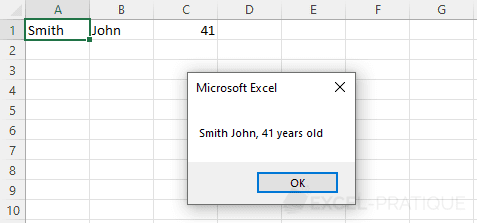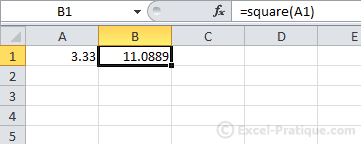# VBA Course: Procedures and Functions

## Public - Private

For the moment, all the procedures that we have created are of the Public type, which means that they are accessible from any module.

``````Sub example()
'Identical to:
Public Sub example()``````

To make a procedure accesible only from within the module, use Private:

``Private Sub example()``

## Launch a procedure from within a procedure

To execute a procedure from within another procedure, simply enter its name.

Here is a very simple example of this:

``````Private Sub warning()
MsgBox "Caution !!!"
End Sub

Sub macro_test()
If Range("A1") = "" Then
warning ' <= execute the procedure "warning"
End If
'etc ...
End Sub``````

Here, when "macro_test" is executed and when A1 has the value "", the "warning" procedure will be executed.

## Arguments

Arguments make it possible to use values from a procedure in a sub procedure (remember that by default, variables are only accessible from the procedure in which they are declared).

``````Private Sub warning(var_text As String)
MsgBox "Caution: " & var_text & " !"
End Sub

Sub macro_test()
If Range("A1") = "" Then
warning "empty cell"
ElseIf Not IsNumeric(Range("A1")) Then
warning "non-numerical value"
End If
End Sub``````An argument has been added to the "warning" procedure, in this case it is the "var_text" variable of type "String":

``Private Sub warning(var_text As String)``

This procedure needs an argument, so we will have to put a value after "warning" to execute it:

``warning "empty cell"``

When there are multiple arguments, these should be separated by commas.

## Optional arguments

By default, if a procedure has arguments, these are mandatory, and if they are not included, the procedure will not execute.

Optional arguments can be added after the mandatory ones using Optional, for example:

``Private Sub dialog_boxes(last_name As String, Optional first_name, Optional age)``

Now this procedure can be executed with or without optional arguments, like this:

``````'Example 1: the last name is displayed:
dialog_boxes last_name1

'Example 2: last name and first name are displayed:
dialog_boxes last_name1, first_name1

'Example 3: last name and age are displayed:
dialog_boxes last_name1, , age1

'Example 4: last name, first name, and age are displayed:
dialog_boxes last_name1, first_name1, age1``````

Arguments must be entered in the correct order.

To test whether an optional argument is present, we will use the IsMissing function. This function is only compatible with certain types of functions (thus Variant), and this is crucial because the type of the optional arguments has not been specified in the declaration (a non declared type is = Variant).

Here is an example using the two snippets of code above:

``````Sub macro_test()

Dim last_name1 As String, first_name1 As String, age1 As Integer

last_name1 = Range("A1")
first_name1 = Range("B1")
age1 = Range("C1")

'Example 1: the last name is displayed:
dialog_boxes last_name1

'Example 2: last name and first name are displayed:
dialog_boxes last_name1, first_name1

'Example 3: last name and age are displayed:
dialog_boxes last_name1, , age1

'Example 4: last name, first name, and age are displayed:
dialog_boxes last_name1, first_name1, age1

End Sub

Private Sub dialog_boxes(last_name As String, Optional first_name, Optional age)

If IsMissing(age) Then 'If the age variable is missing ...

If IsMissing(first_name) Then 'If the first_name variable is missing, only the last name will be displayed
MsgBox last_name
Else 'Otherwise, last name and first name will be displayed
MsgBox last_name & " " & first_name
End If

Else 'If the age variable is present ...

If IsMissing(first_name) Then 'If the first_name variable is missing, last name and age will be displayed
MsgBox last_name & ", " & age & " years old"
Else 'Otherwise, last name, first name, and age will be displayed
MsgBox last_name & " " & first_name & ", " & age & " years old"
End If

End If

End Sub``````

See image below (example 1):## ByRef - ByVal

By default, arguments are of the ByRef type, which means that if a variable is submitted as an argument, its reference will be transmitted. In other words, if the variable is modified in the sub procedure, it will also be modified in the procedure that called the sub procedure.

For example:

``````Sub macro_test()
Dim var_number As Integer
var_number = 30

calcul_square var_number

MsgBox var_number
End Sub

Private Sub calcul_square(ByRef var_value As Integer) 'ByRef does not need to be specified (because it is the default)
var_value = var_value * var_value
End Sub``````

To make this even clearer, here is an explanation of what happens when the macro is launched:

``````var_number = 30
'The initial value of the "var_number" variable is 30

calcul_square var_number
'The sub procedure is launched with "var_number" as an argument

Private Sub calcul_square(ByRef var_value As Integer)
'The "var_value" variable is in some way a shortcut to "var_number", which means that if the "var_value" variable is modified, the "var_number" variable will also be modified (and they don't have to have the same name)

var_value = var_value * var_value
'The value of the "var_value" variable is modified (and therefore the "var_number" is modified as well)

End Sub
'End of sub procedure

MsgBox var_number
'The "var_number" variable was modified, so 900 will now be displayed in the dialog box``````

A second method is to use ByVal.

Unlike ByRef, which transmits the reference (shortcut), ByVal transmits the value, which means that the value submitted as an argument will not be modified.

Here you can see how the code immediately above, and ByVal work:

``````var_number = 30
'The initial value of the variable "var_number" is 30

calcul_square var_number
'The sub procedure is launched with the variable "var_number" as an argument

Private Sub calcul_square(ByVal var_value As Integer)
'The variable "var_value" copies the value of the variable "var_number" (the 2 variables are not linked)

var_value = var_value * var_value
'The value of the variable "var_value" is modified

End Sub
'End of sub procedure (the sub procedure in this example doesn't have any effect at all)

MsgBox var_number
'The variable "var_number" has not been modified, and so 30 will be displayed in the dialog box``````

What you should remember: using ByVal when a variable shouldn't be modified ...

## Functions

The main difference between Sub and Function is the value returned by the function.

Here is a straightforward example:

``````Function square(var_number)
square = var_number ^ 2 'The function "square" returns the value of "square"
End Function

Sub macro_test()
Dim result As Double
result = square(9.876) 'The variable result is assigned the value returned by the fonction
MsgBox result 'Displays the result (the square of 9.876, in this case)
End Sub``````

Functions can be used on a worksheet like any other Excel function.

For example, to obtain the square of the value of A1: